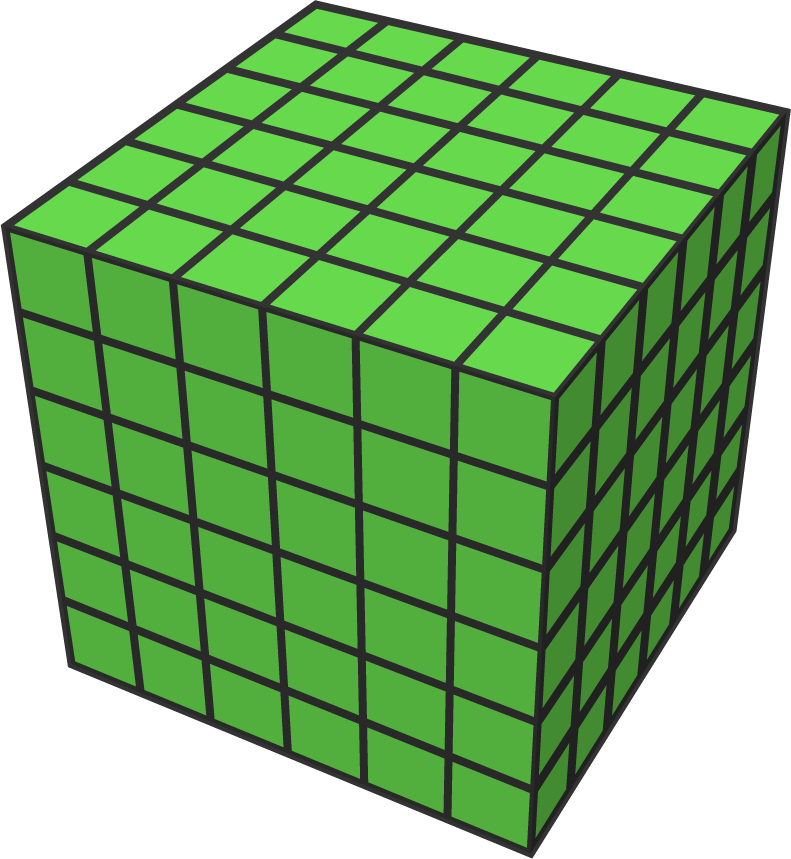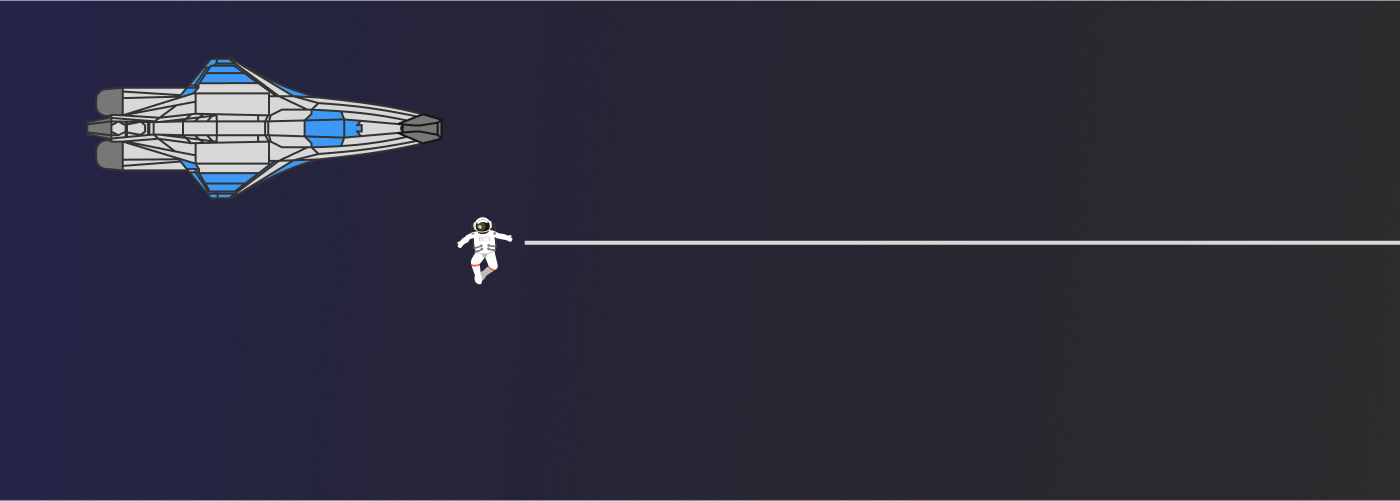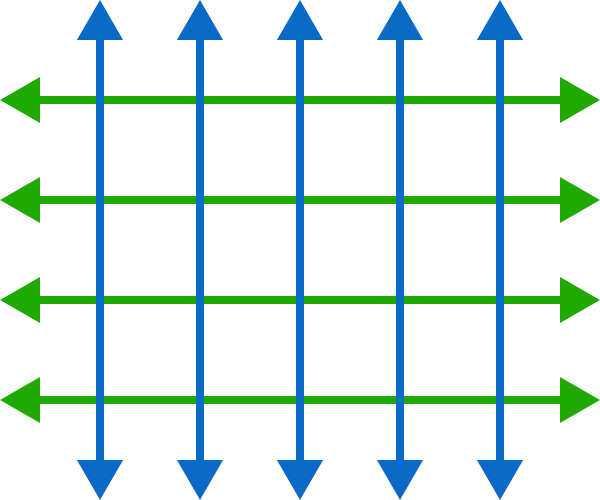# Problems of the Week

Contribute a problem

# 2018-04-16 Intermediate

$1 - \frac{1}{{\color{#3D99F6}2}} + \frac{1}{{\color{#3D99F6}2}} - \frac{1}{{\color{#D61F06}3}} +\frac{1}{{\color{#D61F06}3}} - \frac{1}{{\color{#20A900}4}} + \frac{1}{{\color{#20A900}4}} - \cdots = 1$ This infinite telescoping sum converges to $1,$ partly because all the terms except $1$ get cancelled.

Can we say that the infinite telescoping product below also converges to $1$ for a similar reason?

$\frac{1}{{\color{#3D99F6}2}} \times \frac{{\color{#3D99F6}2}}{{\color{#D61F06}3}} \times \frac{{\color{#D61F06}3}}{{\color{#20A900}4}} \times \frac{{\color{#20A900}4}}{{\color{#624F41}5}}\times \frac{{\color{#624F41}5}}{{\color{#E81990}6}} \times \frac{{\color{#E81990}6}}{7} \times {\cdots } = 1$Consider a $6\times 6\times 6$ cube made up of $216$ small cubes.

How many of these small $1\times 1\times 1$ cubes are visible by rotating the larger cube?

While exploring a nearby interstellar neighborhood, you stumble upon a long metal rod made of aluminum. You use your tape measure to determine it’s $\SI{10}{\centi\meter}$ in diameter and exactly 1 lightyear in length.

Now, you decide to push the rod on one of its ends in the direction of the rod's length. Once you push the rod, you hop in your space ship and take off towards the other end.

Can you get to the other end before the other end starts moving?Assume that your spaceship can travel at speeds up to, but not greater than, the speed of light $c.$

In this diagram, there are twelve $1\times 1,$ six $2\times 2,$ and two $3\times 3$ squares for a total of 20 squares.What is the least number of lines needed in total to have exactly 100 squares?

$\begin{cases} x^{2}+y^{2}+z^{2}=\frac{1}{4} \\\\ x^{3}+y^{3}+z^{3}=\frac{1}{6} \end{cases}$

How many real solutions $(x, y, z)$ does the system above have?

×